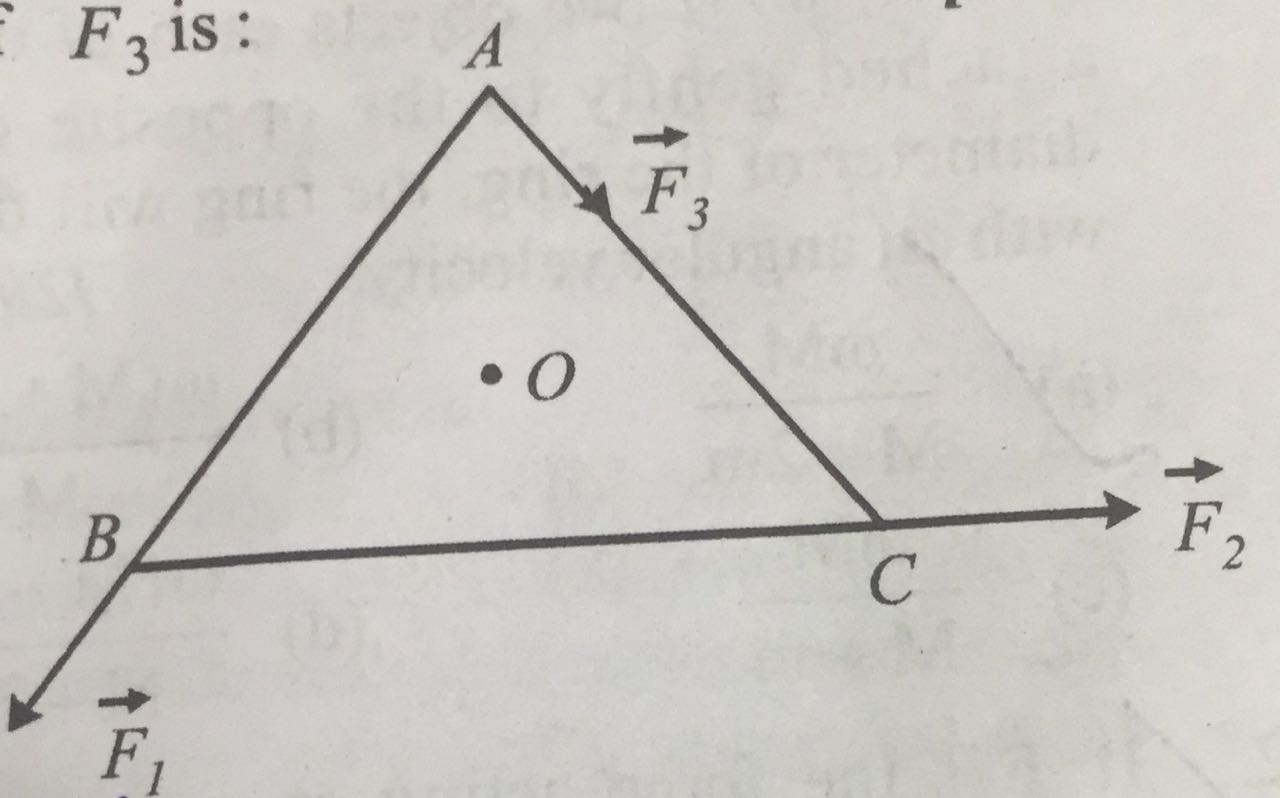# ABC is an equilateral triangle with O as its centre. represent three forces acting along the sides AB, BC and AC respectively. If the total torque about O is zero the magnitude ofOption 1) Option 2) Option 3) Option 4)

As discussed in

Equilibrium -

Translational equilibrium

Rotational equilibrium

-

Option 1)

This option is correct.

Option 2)

This option is incorrect.

Option 3)

This option is incorrect.

Option 4)

This option is incorrect.

Exams
Articles
Questions